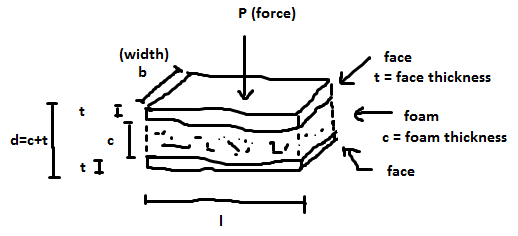Sandwich
b (width) = mm
c (core thickness) = mm
t (face thickness) = mm
L (beam length) = mm

Face data (for example GFRP) Ef (elastic Young's/tensile modulus face) = GPa
σfy (tensile strength yield face) = MPa

Core data (for example XPS foam) Materials:
Es (elastic Young's/tensile modulus core solid) = GPa
σfs (tensile strength yield core solid) = MPa
ρs (density core solid) = kg/m3
ρc (density core foam) = kg/m3

Face Yield = N
Face Wrinkle = N
Core Shear = N
Deflection = mm/N